;nbsp

Hi parents and students! This is an introduction to what you’ll be needing to know by the end of the first Calculus semester. My courses cover all of the common core requirements (the syllabus for semester 1 is here, and semester 2 is here!) and prepare my students to think critically. Not only do I want your student to know the material in my class, I want them to succeed in all future classes and opportunities. Please try out this sample test to see what your child will be learning this semester!

Name

This is a required question

Email

Must be a valid email address
This is a required question1. Determine the value of this limit.
This is a required question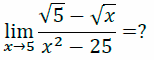2. Determine the value of this limit.
This is a required question3. Determine the value of A so as to insure that this function is everywhere continuous.
This is a required question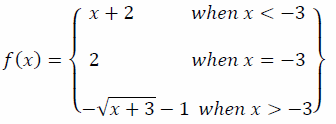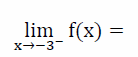4a. Identify the value of this limit.
This is a required question4b. Identify the value of this limit.
This is a required question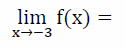4c. Identify the value of this limit.
This is a required question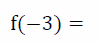4d. Identify the value of this expression.
This is a required question

5. Find the instantaneous rate of change of f(x) = 2x² + 5x at x = 3 using the limit definition of the instantaneous derivative.
This is a required question

6a. Using the formal definition of the derivative, find the slope of the tangent line to the curve given by f(x) = 1/(x – 6).
This is a required question

6b. Find the slope of the normal line to the curve given by f(x) = 1/(x – 6).
This is a required question

6c. Find the slope of the normal line to the curve given by f(x) = 1/(x – 6) at x = 0.
This is a required question

7. Which of the following is (are) correct representation(s) of the derivative of f(x) evaluated at a?
All correct answers must be selected. No partial credit.
This is a required question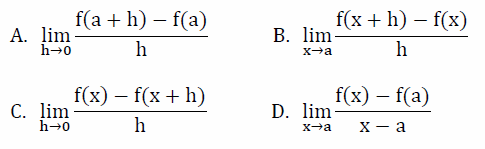8. Find f ‘ (θ) when f(θ) = cos(θ)cot(θ)
This is a required question

9. Find g'(t) when g(t) = 3t/(t – 4)
This is a required question

10a. Find the slope of the tangent line to the curve given by f(x) = (x³ + 7)(√2x)³
This is a required question

10b. Find the equation of the tangent line (at x = 0) to the curve given by f(x) = (x³ + 7)(√2x)³
This is a required question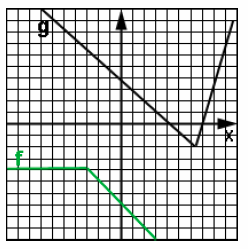11a. Using the functions f(x) and g(x) from the above graph, find f ’(2).
This is a required question

11b. Using the functions f(x) and g(x) from the above graph, find g ’(2).
This is a required question

11c. Using the functions f(x) and g(x) from the adjacent graph, find p ’(2) where p(x) = 3f(x)g(x).
This is a required question

12. Find h ’(x) given h(x) = 9x³√x⁴ + 7
This is a required question

13. Find f ’(x) where f(x) = sin( tan(x) ).
This is a required question

14a. Find dy/dx when y = -4cos(11x).
This is a required question

14b. Find d²y/dx² when y = -4cos(11x).
This is a required question

## An object is moving rectilinearly in time with velocity given by v(t) = 6t – t² with units m/s. The position at t = 0 is 5 meters.

15a. Find the position function of time.
This is a required question

15b. What are the appropriate units for 15a?
This is a required question

15c. An object is moving rectilinearly in time with velocity given by v(t) = 6t – t² with units m/s. Assuming that position at t = 0 is 5 meters, find the acceleration function of time with appropriate units.
This is a required question

15d. What are the appropriate units for 15c?
This is a required question

16. Differentiate implicity to find y’ where 4x³y = 2x + sin(y)
This is a required question

## Assume f(0) = 2, f'(0) = -1, f”(0) = 1, and g(x) = sec(3x)f(x). What is the equation of the line tangent to g(x) at x = 0.

17a. Identify the correct equation for g'(x).
This is a required question

17b. What is the slope of the tangent line of g(x) at x = 0.
This is a required question

17c. What is the equation of the tangent line of g(x) at x = 0.
This is a required question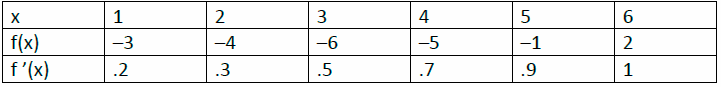18a. Find g'(x) where g(x) = ( f(2x) )²
This is a required question

18b. Use the data in this chart to find g'(3) where g(x) = ( f(2x) ) ²
This is a required question

19. A large cube is losing volume at the rate of .2 m³/sec while still maintaining its cubical shape. What is the rate of change of the sides when the sides of the cube measure 2 meters?
This is a required question

20. Consider the function f(x) = cos(x) + x over the interval [0, 1]radians. Show that the conditions of the Mean Value Theorem are met over this interval and then find the value of ξ predicted by the theorem.
This is a required question

## For each x value below, state if it is a critical value or not.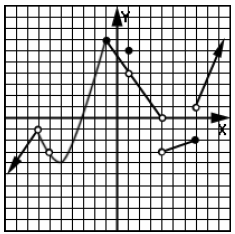21a. x = -7
This is a required question

21b. x = -6
This is a required question

21c. x = -5
This is a required question

21d. x = -1
This is a required question

21e. x = 1
This is a required question

21f. x = 4
This is a required question

21g. x = 7
This is a required question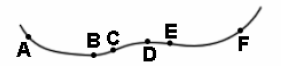22. Which of the points in the graph of f(x) shown above have both a negative first derivative and also a negative second derivative value?
This is a required question

23. A rectangular beam is cut out of a circular log of radius R. What is the maximum area of the log?
This is a required question

24. What is the derivative of f(x) = ³√(4^(3x) + log₄x) ?
This is a required question

25. Find f ’(x) where f(x) = arcsin(2x³/5).
This is a required question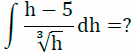26. Find the value of the indefinite integral above.
This is a required question

27. What is f(x) when f ’(x) = 1/cos²(x) and f(2π/3 radians) = 2?
This is a required question

## Find f(x) when f ’’(x) = x + sin(5x) , f ’(π/6 radians) = 0, and f(0 radians) = 1.

28a. Find f ‘ (x) by taking the indefinite integral of f’’(x)
This is a required question

28b. Find C₁
This is a required question

28c. Find f(x) by taking the indefinite integral of f'(x)
This is a required question

28d. Find C₂ and indentify the final formula for f(x).
This is a required question

This is a required question